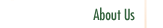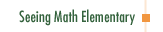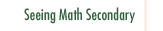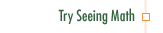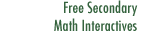Data Analysis and Probability:
Using Data to Make Predictions

In Data Analysis and Probability: Using Data to Make Predictions, we present ways to support NCTM standards for grades 3-5 that invite students to collect, analyze, and make predictions from data. The teaching case presents two lessons taught to a fifth grade class. The first lesson explores mathematical fairness. The second lesson highlights the relationship between sample size and accuracy of predictions about a whole population.

Participants in this course:

• Explore the difference between social fairness and mathematical fairness.
• Examine how probability relates to causality.
• Understand relations among sample size, data collection, and accurate predictions.
• Develop strategies that support student thinking, reflection, and dialogue.
• Explore how graphic representations can indicate accuracy of data measurements.
• Develop ways to guide students from faulty to sound mathematical concepts.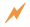Click to Get Seeing Math™ ElementaryCourse Listing Effective Questioning in the Mathematics Classroom Foundations of EffectiveMathematics Teaching Formative Assessment in the Mathematics Classroom Number & Operations: Division with Remainders Number & Operations: The Magnitude of Fractions Number & Operations: Broken Calculator Geometry: 2D and 3D Figures Geometry: Calculating Area of a Triangle Data Analysis and Probability: Using Data to Make Predictions Data Analysis and Probability: Measures of Center Pre-Algebra: Pan Balance Equations Pre-Algebra: Patterns and Functions